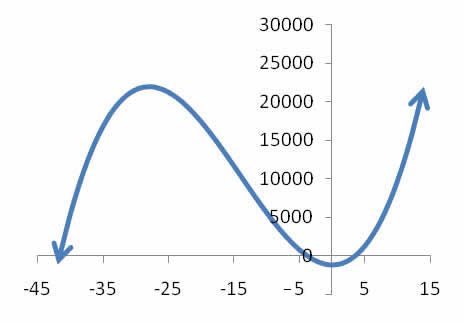# Rocket Design

## A rocket consists of a right circular cylinder of height 28 meters surmounted by a cone whose diameter is equal to its height. Determine the radius of the rocket if it is to have a total volume of 1000 cubic meters.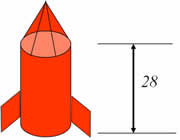## The volume of the cylinder section is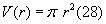## The volume of the cone section is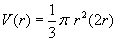## Summing the two volumes results in a cubic polynomial for the total volume.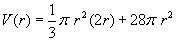## We want a rocket volume of 1000 cubic meters, so we need to solve for r when V = 1000. Putting in this value and subtracting 1000 from both sides gives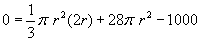## Our knowledge of cubic polynomials implies that we either have 1 real solution and 2 complex solutions or 3 real solutions where we will need to determine which of the 3 solutions is best. Graphing the right side of the equation to determine the real zeros will give the radius of the cone section that produces a total volume of 1000.